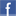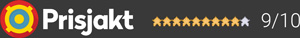#### Inga produkter tillagda!

Lägg en produkt i varukorgen så ser det lite roligare ut här ;)

#### Varukorg

0 kr# DevialetPHANTOM II 98 DB OPÉRA DE PARIS

18 990 krLeverans 2-5 dagar ()
Fri frakt!

## Fler bilder

Monumental, wherever you're seated.
98 dB SPL. 18Hz – 21kHz. 400 Watts RMS.

The master gilders at Ateliers Gohard finished the Phantom’s gold leaf side plates following time-honored methods. Each gold leaf is applied by hand to create a unique patina. And these heritage techniques demand absolute perfection—once the leaf has been applied, it cannot be removed. Bearing the Phantom II 98 dB Opéra de Paris | Devialet signature, the side plates are gilded with Moon Gold, a precious and stainless palladium-yellow gold alloy.

Garanti i 24 månaderDela på Facebook

#### Frågor? Ring 031-241600eller maila //<![CDATA[ var l=new Array(); l = '>'; l = 'a'; l = '/'; l = '<'; l = '|109'; l = '|111'; l = '|99'; l = '|46'; l = '|110'; l = '|114'; l = '|97'; l = '|108'; l = '|108'; l = '|97'; l = '|107'; l = '|100'; l = '|117'; l = '|106'; l = '|108'; l = '|64'; l = '|111'; l = '|102'; l = '|110'; l = '|105'; l = '>'; l = '"'; l = '|109'; l = '|111'; l = '|99'; l = '|46'; l = '|110'; l = '|114'; l = '|97'; l = '|108'; l = '|108'; l = '|97'; l = '|107'; l = '|100'; l = '|117'; l = '|106'; l = '|108'; l = '|64'; l = '|111'; l = '|102'; l = '|110'; l = '|105'; l = ':'; l = 'o'; l = 't'; l = 'l'; l = 'i'; l = 'a'; l = 'm'; l = '"'; l = '='; l = 'f'; l = 'e'; l = 'r'; l = 'h'; l = ' '; l = 'a'; l = '<'; for (var i = l.length-1; i >= 0; i=i-1) { if (l[i].substring(0, 1) === '|') document.write("&#"+unescape(l[i].substring(1))+";"); else document.write(unescape(l[i])); } //]]>